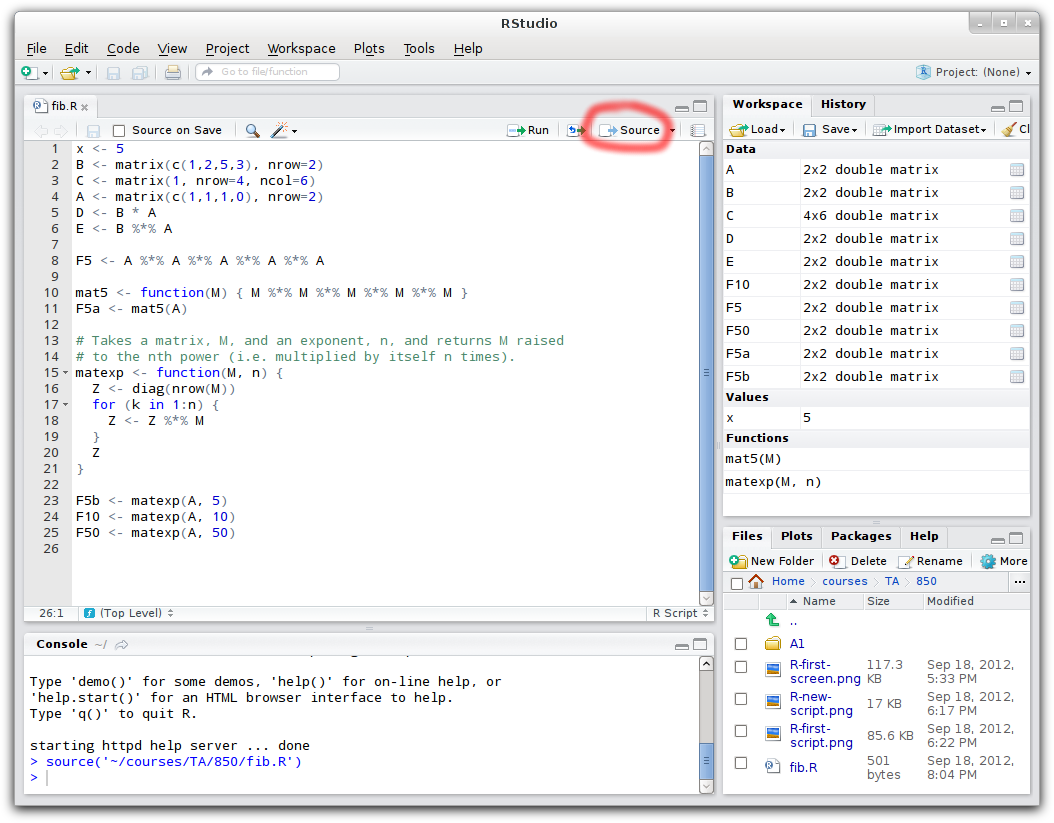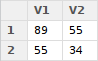# A First Program: Fibonacci matrix

As a first program, let’s do some basic matrix calculations.  What I want to do (in R) is define the matrix $$A \equiv \left(\begin{array}{cc}1 & 1 \\ 1 & 0 \end{array}\right)$$ and then calculate $$A^5$$, $$A^{10}$$ and $$A^{50}$$.  (For the mathematically curious, this matrix, when multiplied by itself, yields the Fibonacci sequence: $$A^n = \left(\begin{array}{cc}F_{n+1}&F_n\\F_n&F_{n-1}\end{array}\right)$$, where $$F_n$$ is the nth Fibonacci number.)

The assignment operator in R is two characters: ‘<-‘.  It is probably most intuitive to think of this as an arrow, “pointing” one value at a particular place.  For example,

x <- 5

assigns the value 5 to variable x.  But we want to assign a matrix, not a single value.  There are a few ways to create a matrix in R, with the built-in matrix() function being the most common.  matrix() takes a list of values and at least one of the number of rows or columns of the matrix.  For a list of fixed values, you often want to use the c() function, e.g. c(1,2,5,3).  So, for example, let’s add another line to our code:

B <- matrix(c(1,13,2,15), nrow=2)

This assigns the matrix $$\left(\begin{array}{cc}1&2\\13&15\end{array}\right)$$ to the variable B.  Note that we didn’t have the specify the number of columns: R can figure that out itself.  Also note that R loads values in column-order by default.  If you do specify both nrow and ncol, R has a nifty feature: it will repeat your value (or values) to fill out the matrix.  Thus the following creates a 4×6 matrix of all 1s:

C <- matrix(1, nrow=4, ncol=6)

Anyway, let’s assign the matrix we’re actually interested in to A:

A <- matrix(c(1,1,1,0), nrow=2)

Now we need to do the matrix multiplication.  In R, ‘*’ is used for element-by-element multiplication, so the following:

D <- B * A

would actually calculate $$\left(\begin{array}{cc}b_{11}a_{11} & b_{12}a_{12} \\ b_{21}a_{21} & b_{22}a_{22}\end{array}\right)$$, which is not the matrix multiplication that we want. Instead, matrix multiplication uses the ‘%*%’ operator:

E <- B %*% A

So, to calculate our intended $$A^5$$ (and save the result in variable ‘F5’), we want:

F5 <- A %*% A %*% A %*% A %*% A

We can do better, however, by defining a function to carry out the matrix exponentiation. In R, a function is something that you assign to a variable just like a number, then call it by referring to the variable with brackets after it. Let’s create a function named ‘mat5’ that takes a matrix and gives back the matrix multiplied by itself 5 times:

mat5 <- function(M) { M %*% M %*% M %*% M %*% M }

Now, instead of the F5 <- … line above, I could write:

F5a <- mat5(A)

to get the same result.

As a general programming technique, any time you find yourself repeating a set of calculations (with perhaps just a few variable changes), write a function!  You will save yourself a substantial amount of time.  I can’t express to you the number of times people who haven’t got their heads around using functions will write, say, 5 lines of code, then copy and paste those 5 lines several times (let’s say 6 times), changing something small in each block of 5 lines.  Then, when they run the code, they find a mistake in the copy-and-pasted lines.  Now, instead of having to fix 5 lines, you sudden have to fix 30 lines!  Make yourself use functions a few times just to get used to them: you’ll save yourself time, aggrevation, and you’ll make your code much easier to follow, both for yourself and for others.

Back to our ‘mat5’ function above, then: instead of making it multiply itself 5 times, let’s make the function take two arguments: a matrix and a number of times to multiply the loop.  Let’s also write a comment to ourselves, so that when we look back at this function later, we know what it does (or, if it isn’t working properly, we know what we intended it to do):

# Takes a matrix, M, and an exponent, n, and returns M raised
# to the nth power (i.e. multiplied by itself n times).
matexp <- function(M, n) {
Z <- diag(nrow(M));
for (k in 1:n) {
Z <- Z %*% M;
}
Z
}

There are a few things to explain here:

• # begins a comment.  It can take up the whole line, as above, or part of a line, such as:
x <- 5 # Assign my favourite number to my favourite variable!
• nrow(M) returns the number of rows of the matrix M.
• diag(n) is the identity matrix of size nxn.  (diag() has other uses, too: for example, diag(c(1,2,8,3)) gives you the 4×4 matrix {tex inline}left(begin{array}{cccc}1&0&0&0\0&2&0&0\0&0&8&0\0&0&0&3end{array}right){/tex}.  If you pass diag() a matrix, it extracts the elements on the diagonal of the matrix: for example, with our A matrix above, diag(A) would give us c(1,0).)
• ‘for (var in list) { … }’ is called a “for loop”: it runs the code … once for each element of list, setting that element to the variable var.  So, if n is equal to 4, the for loop above repeats the matrix multiplication once with k equal to 1, then 2, then 3, then 4.
• The Z at the very end of the function is telling R what the value returned by the function will be.

Putting this all together in words, the function does this: Given a matrix M and exponent n, set Z to an identity matrix of the same size as M, then, repeating n times in all, set Z to itself multiplied by M.  Finally, return Z.

To summarize, you should have a program that looks like the following:

x <- 5
B <- matrix(c(1,2,5,3), nrow=2)
C <- matrix(1, nrow=4, ncol=6)
A <- matrix(c(1,1,1,0), nrow=2)
D <- B * A
E <- B %*% A
F5 <- A %*% A %*% A %*% A %*% A
mat5 <- function(M) { M %*% M %*% M %*% M %*% M }
F5a <- mat5(A)
# Takes a matrix, M, and an exponent, n, and returns M raised
# to the nth power (i.e. multiplied by itself n times).
matexp <- function(M, n) {
Z <- diag(nrow(M))
for (k in 1:n) {
Z <- Z %*% M
}
Z
}
F5b <- matexp(A, 5)
F10 <- matexp(A, 10)
F50 <- matexp(A, 50)

I added the last 3 lines to make use of the new function.  If you haven’t done so already, copy and paste the above into an empty R script (not into the console).  You’ll notice that R highlights values, functions, and comments in various colours to help you read your code.  Save this code to some suitable location on your computer.  I called mine ‘fib.R’.

So far, however, we haven’t actually told R to actually run our program, we just wrote it into a (text) file named fib.R.  To actually run your whole file from top to bottom, use the “Source” button on the right hand side, just above the first line of your R program. Once you do, you should see something that looks like the following.  Notice that the list of variables in the top right pane, which was previously empty, now has all our variables (A, B, C, F5, etc.) listed.Clicking on the variables will display their values.  For example, when you click on F10, you should get:(RStudio opens this variable display as a separate tab in the same space as your R program: close the ‘F10’ tab, or click on the ‘fib.R’ tab to go back to your file).

(If you feel like verifying the numbers, here is a list of the first 300 Fibonacci numbers).

If you’ve followed along this far, you’re doing well; the next few pages will discuss in more detail the various functions you’ll need to use to accomplish various tasks in R.

Before getting any further, let’s discuss using R’s built-in help pages.